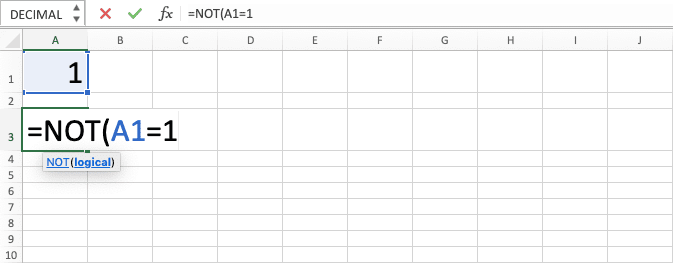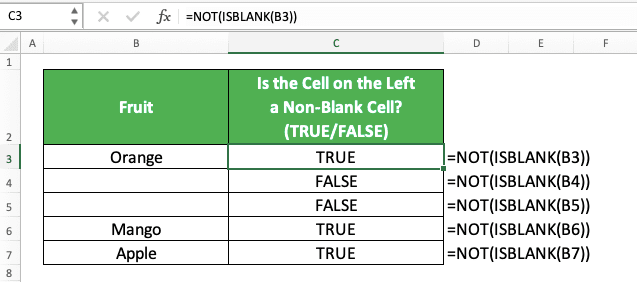How to Use the NOT Function in Excel: Usabilities, Examples and How to Write It - Compute Expert

# How to Use the NOT Function in Excel: Usabilities, Examples and How to Write It

Home >> Excel Tutorials from Compute Expert >> Excel Formulas List >> How to Use the NOT Function in Excel: Usabilities, Examples and How to Write It

In this tutorial, you will learn how to use the NOT function in excel completely.

When working based on logic in excel, we may sometimes want to overturn the logic value of a logic condition. In other words, we want TRUE to become FALSE and FALSE to become TRUE.

We might need that kind of thing so we can get the result we want from our data processing correctly. If we know how to use the NOT formula in excel, then we can do the logic value overturning process easily.

Want to master the way to use NOT so it can support you when you work with logic conditions in excel? Read this tutorial until its last part.

Disclaimer: This post may contain affiliate links from which we earn commission from qualifying purchases/actions at no additional cost for you. Learn more

## What is the NOT Function in Excel?

NOT is an excel function that helps you to get the opposite of the logic value you input into it.

## NOT Usability

You can use NOT to get TRUE from FALSE and FALSE from TRUE in excel. We usually input the TRUE or FALSE logic value to NOT in the form of a logic condition.

## NOT Result

The result from NOT is a TRUE or FALSE logic value. It is the opposite of the logic value you input into it.

## Excel Version from Which We Can Use NOT

We can start using NOT in excel since excel 2003.

## The Way to Write It and Its Input

Here is the general writing form of NOT in excel.

= NOT ( logic_value )

We only need to input the logic value we want to overturn to NOT. The logic value we input is usually in the form of a logic condition.

## Example of Its Usage and Result

Here is an implementation example of NOT in excel.As you can see here, we can overturn the logic value we have with NOT easily. Just input our logic value to NOT, press enter, and we will get its opposite immediately.

This also goes the same with a logic condition you want to overturn the logic value of with NOT. Just input the logic condition to the NOT you write to get your result.

## Writing Steps

Want to know the detailed writing steps of a NOT formula in excel? Read below!

1. Type an equal sign ( = ) in the cell where you want to put the NOT result2. Type NOT (can be with large and small letters) and an open bracket sign after =3. Input the logic condition/value which TRUE/FALSE you want to overturn4. Type a close bracket sign5. Press Enter
6. Done!## How to Identify Cells that Don’t Have Some Specific Content with NOT: IF NOT

Need to identify your cells that do not contain a particular content? You can combine IF and NOT formulas for a clearer identification result of that.

Here is the general writing form of the two formulas combination for the purpose.

= IF ( NOT ( cell = specific_content ) , “No” , “Yes” )

Input the logic condition where your cell coordinate is equal to the cell content you want to identify to NOT. From there, you will get TRUE if the cell doesn’t contain the content and FALSE if it does. Place the NOT formula in the logic condition input part of your IF.

After that, you can input the IF result if the cell doesn’t contain the content or if it does. In the writing form above, we identify it with the word “No” and “Yes”. You can obviously change the identification result according to your preference.

Here is an implementation example of the IF and NOT combination for a deeper understanding of its concept.In the example, we want to identify whether our cells contain “Apple” or not. As you can see, we can identify it easily by using the IF and NOT formulas combination.

We input the cell where we want to identify the “Apple” content with an equal symbol and “Apple” text to NOT. After that, we input the “No” and “Yes” texts as the “Apple” content identification results in our IF.

This writing is as we have discussed before regarding the way to combine the IF and NOT formulas. As a result of that, we get the “Apple” content identification result for all of our cells!

## How to Identify Non-Blank Cells: NOT ISBLANK

Need to identify whether a cell is not blank or blank in excel? You can combine the writing of NOT and ISBLANK formulas for that.

ISBLANK is a formula you can use to identify whether a cell is blank or not. By combining it with NOT, you can get TRUE if the cell is a non-blank cell instead.

Here is the general writing form of the NOT and ISBLANK formulas in excel for the non-blank cel lidentification purpose.

= NOT ( ISBLANK ( cell ) )

And here is its implementation example.If you want to get an identification result other than TRUE or FALSE, you can combine both formulas with an IF. Just put the NOT and ISBLANK writing in the IF logic condition input part to combine them.

## Exercise

After you have learned how to use NOT in excel, let’s do an exercise to deepen your understanding.

### Instructions

1. Mark all data in the most left column with “Not Number” and “Number” in column no 1! Combine IF, NOT, and ISNUMBER formulas to help you do the marking process!
2. Mark all data in the most left column with “Not Text” and “Text” in column no 2! Combine IF, NOT, and ISTEXT formulas to help you do the marking process!
3. Mark all data in the most left column with “Not Error” and “Error” in column no 3! Combine IF, NOT, and ISERROR formulas to help you do the marking process!

As NOT result is a logic value (TRUE or FALSE), we usually use it with a formula that utilizes a logic value input (like IF).

Related tutorials you should learn from:

Get updated excel info from Compute Expert by registering your email. It's free!